JEE  >  Irodov Solutions: Mechanical Oscillations- 6

# Irodov Solutions: Mechanical Oscillations- 6 | I. E. Irodov Solutions for Physics Class 11 & Class 12 - JEE

 1 Crore+ students have signed up on EduRev. Have you?

Q.66. A coil of inductance L connects the upper ends of two vertical copper bars separated by a distance l. A horizontal conducting connector of mass m starts falling with zero initial velocity along the bars without losing contact with them. The whole system is located in a uniform magnetic field with induction B perpendicular to the plane of the bars. Find the law of motion x (t) of the connector.

Ans. As the connector moves, an emf is set up in the circuit and a current flows, since the emf is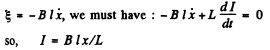provided x is measured from the initial position.

We then have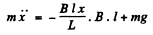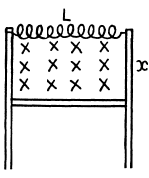for by Lenz’s law the induced current will oppose downward sliding. Finally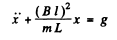on putting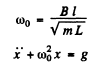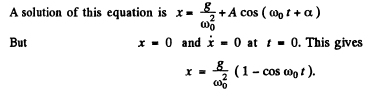Q.67. A point performs damped oscillations according to the law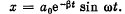Find: (a) the oscillation amplitude and the velocity of the point at the moment t = 0; (b) the moments of time at which the point reaches the extreme positions.

Ans. We are given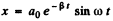(a) The velocity of the point at t = 0 is obtained from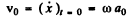The term "oscillation amplitude at the moment t = 0" is meaningless. Probably the implication is the amplitude for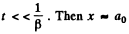sin ωt and amplitude is ao.

(b)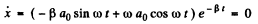when the displacement is an extremum. Then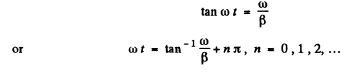Q.68. A body performs torsional oscillations according to the law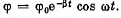Find:

(a) the angular velocity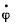and the angular acceleration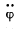of the body at the moment t = 0; (b) the moments of time at which the angular velocity becomes maximum.

Ans.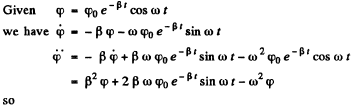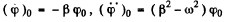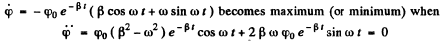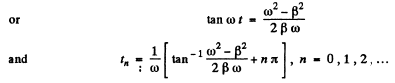Q.69. A point performs damped oscillations with frequency ω and damping coefficient   according to the law (4.1b). Find the initial amplitude a, and the initial phase a if at the moment t = 0 the displacement of the point and its velocity projection are equal to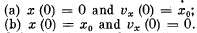Ans.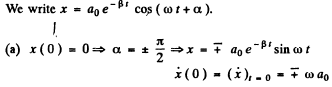Since a0 is + ve, we must choose the upper sign if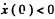and the lower sign if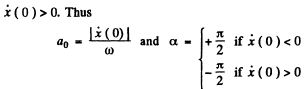(b)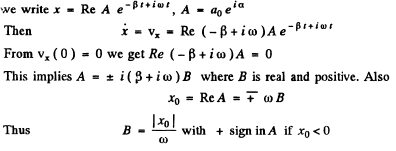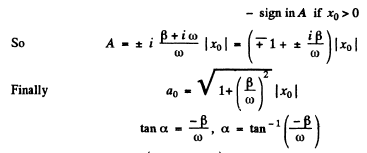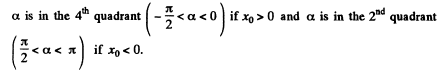Q.70. A point performs damped oscillations with frequency ω = = 25 s-1. Find the damping coefficient l if at the initial moment the velocity of the point is equal to zero and its displacement from the equilibrium position is η = 1.020 times less than the amplitude at that moment.

Ans.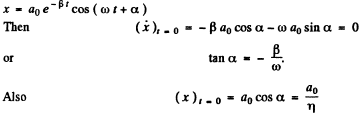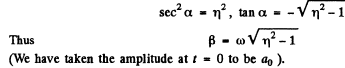Q.71. A point performs damped oscillations with frequency ω and damping coefficient β. Find the velocity amplitude of the point as a function of time t if at the moment t = 0
(a) its displacement amplitude is equal to a0;
(b) the displacement of the point x (0) = 0 and its velocity pro- jection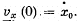Ans.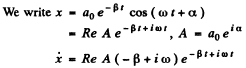Velocity amplitude as a function of time is defined in the following manner. Put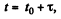then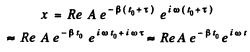for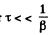This means that the displacement amplitude around the time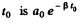and we can say that the displacement amplitude at time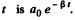Similarly for the velocity amplitude.
Clearly

(a) Velocity amplitude at time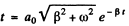Since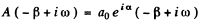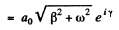where y is anotner constant

(b)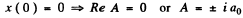where a0 is real and positive.

Also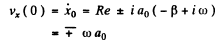Thus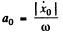and we take - ( + ) sign if x0 is negative (positive). Finally the velocity amplitude is obtained as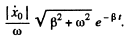Q.72. There are two damped oscillations with the following periods T and damping coefficients β: T1 =  0.10 ms, β1  = 100s-1  and T2  = 10ms, β2  = 10s-1. Which of them decays faster?

Ans. The first oscillation decays faster in time. But if one takes the natural time scale, the period T for each oscillation, the second oscillation attenuates faster during that period.

Q.73. A mathematical pendulum oscillates in a medium for which the logarithmic damping decrement is equal to 20  = 1.50. What will be the logarithmic damping decrement if the resistance of the medium increases n = 2.00 times? How many times has the resistance of the medium to be increased for the oscillations to become impossible?

Ans. By definition of the logarithemic decrement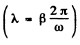we get for the original decrement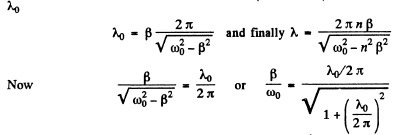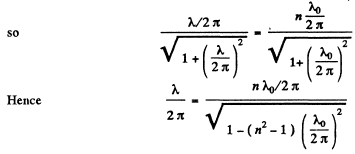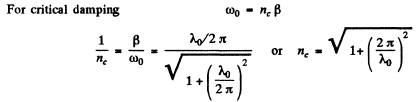Q.74. A deadweight suspended from a weightless spring extends it by Δx = 9.8 cm. What will be the oscillation period of the dead Weight when it is pushed slightly in the vertical direction? The logarithmic damping decrement is equal to λ = 3.1.

Ans.  The Eqn of the dead weight is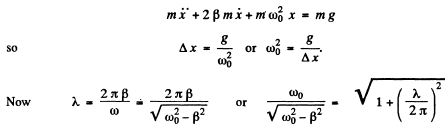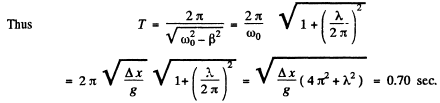Q.75. Find the quality factor of the oscillator whose displacement amplitude decreases η = 2.0 times every n = 110 oscillations.

Ans. The displacement amplitude decrease η times every n oscillations. Thus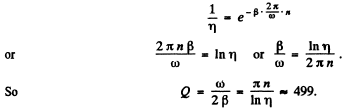Q.76. A particle was displaced from the equilibrium position by a distance l = 1.0 cm and then left alone. What is the distance that the particle covers in the process of oscillations till the complete stop, if the logarithmic damping decrement is equal to λ = 0.020?

Ans.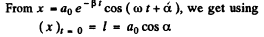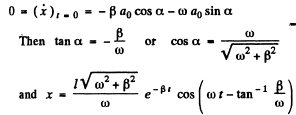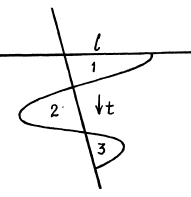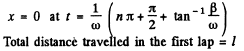To get the maximum displacement in thfe second lap we note that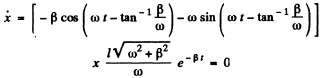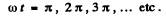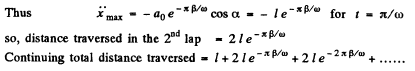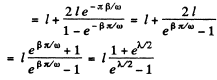where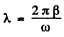, is the logarithemic decrement Substitution gives 2 metres.

Q.77. Find the quality factor of a mathematical pendulum l = = 50 cm long if during the time interval δ = 5.2 min its total mechanical energy decreases η = 4.0.10 times.

Ans. For an undamped oscillator the mechanical energy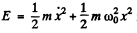is conserved. For a damped oscillator.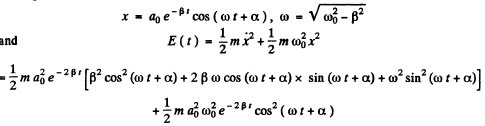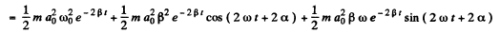If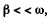then the average of the last two terms over many oscillations about the time t will vanish and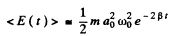and this is the relevant mechanical energy.

In time δ this decreases by a factor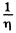so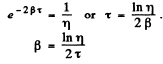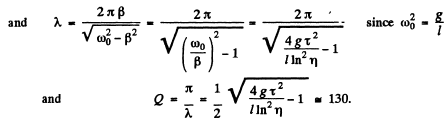Q.78. A uniform disc of radius R = 13 cm can rotate about a horizontal axis perpendicular to its plane and passing through the edge of the disc. Find the period of small oscillations of that disc if the logarithmic damping decrement is equal to λ = 1.00.

Ans. The restoring couple is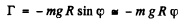The moment of inertia is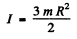Thus for undamped oscillations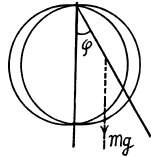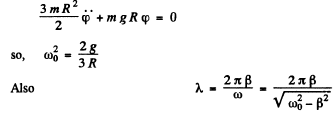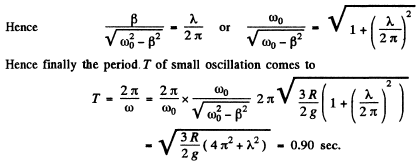Q.79. A thin uniform disc of mass m and radius R suspended by an elastic thread in the horizontal plane performs torsional oscillations in a liquid. The moment of elastic forces emerging in the thread is equal to N = αφ, where a is a constant and IT is the angle of rotation from the equilibrium position. The resistance force acting on a unit area of the disc is equal to F1 = ηv, where is a constant and η is the velocity of the given element of the disc relative to the liquid. Find the frequency of small oscillation.

Ans. Let us calculate the moment G1 of all the resistive forces on the disc. When the disc rotates an element (rdrdθ) with coordinates (r,θ) has a velocity r, where φ is the instantaneous angle of rotation from the equilibrium position and r is measured from the centre. Then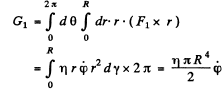Also moment of inertia =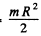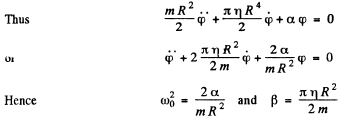and angular frequency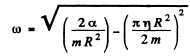Note:- normally by frequency we mean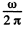Q.80. A disc A of radius R suspended by an elastic thread between two stationary planes (Fig. 4.24) performs torsional oscillations about its axis 00'. The moment of inertia of the disc relative to that axis is equal to I, the clearance between the disc and each of the planes is equal to h, with h << R. Find the viscosity of the gas surrounding the disc A if the oscillation period of the disc equals T and the logarithmic damping decrement, λ.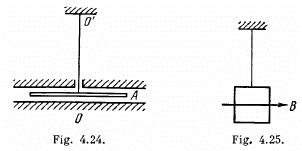Ans. From the law of viscosity, force per unit area =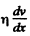so when the disc executes torsional oscillations the resistive couple on it is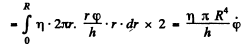(factor 2 for the two sides of the disc; see the figure  in the book) where φ is torsion. The equation of motion is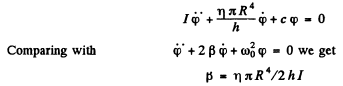Now the logarithmic decrement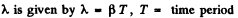Thus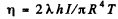The document Irodov Solutions: Mechanical Oscillations- 6 | I. E. Irodov Solutions for Physics Class 11 & Class 12 - JEE is a part of the JEE Course I. E. Irodov Solutions for Physics Class 11 & Class 12.
All you need of JEE at this link: JEE

111 docs

## I. E. Irodov Solutions for Physics Class 11 & Class 12

111 docs

Track your progress, build streaks, highlight & save important lessons and more!(Scan QR code)

,

,

,

,

,

,

,

,

,

,

,

,

,

,

,

,

,

,

,

,

,

;